# Exercise 6.5 The-Triangle-and-its-Properties- NCERT Solutions Class 7

Go back to  'The Triangle and its Properties'

## Chapter 6 Ex.6.5 Question 1

$$PQR$$ is a triangle right angled at $$P.$$ If $$PQ$$ $$=\rm{}\,10\, cm$$ and $$PR$$ $$= \rm{}\,24\, cm,$$ find $$QR.$$

### Solution

What is Known?

$$PQR$$ is a triangle right angled at $$P$$ and the length of two sides $$PQ$$ $$=\rm{}\,10\,cm$$ and $$PR$$ $$= \rm{}\,24\, cm.$$

What is unknown?

Length of one side of the triangle.

Reasoning:

This question is straight forward, as it is given in the question that $$PQR$$ is a right- angled triangle and it is right angled at $$P.$$ So, we can apply Pythagoras theorem here, if it is right angled at $$P$$ then the side opposite to $$P$$ will be the hypotenuse of the triangle i.e.$$QR$$ and the other side is given $$PQ$$ $$= \rm{}\,10\,cm$$ and $$PR=24$$. Now, by applying Pythagoras theorem i.e. in a right-angled triangle, the square of hypotenuse is equal to the sum of square of other two sides, we can find $$QR.$$ For better visual understanding draw a right-angled triangle which is right angled at $$Q$$ and consider the side opposite to it $$PR$$ as hypotenuse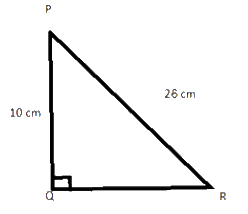Steps:

Given, $$PQ$$ $$= \rm{}\,10\,cm,$$ $$PR$$ $$= \rm{}\,24\,cm$$ and $$QR =?$$

By applying Pythagoras theorem in triangle $$PQR,$$ we get

\begin{align} \left( {{\rm{Hypotenuse}}} \right)^2 & \!=\! \left[ \begin{array}{l} \left( {{\rm{Perpendicular}}} \right)^2 \!+\! \\ \left( {{\rm{Base}}} \right)^2 \\ \end{array} \right] \\ QR^2 &\!=\! \left( {{\rm{PQ}}} \right)^2 \!+\! \left( {{\rm{PR}}} \right)^2 \\ QR^2 &\!=\! \left( {{\rm{10}}} \right)^2 \!+\! \left( {{\rm{24}}} \right)^2 \\ QR^2 &\!=\! 100 \!+\! 576 \\ QR^2 &\!=\! 676 \\ QR &\!=\! 26\;{\rm{cm}} \\ \end{align}

Thus, $$QR$$ is equal to $$26$$ cm

Useful Tip:

Whenever you encounter problems of this kind, it is best to think of the Pythagoras theorem for right angled triangle.

## Chapter 6 Ex.6.5 Question 2

$$ABC$$ is a triangle right angled at $$C.$$ If $$AB$$ $$= \rm{}25\,cm$$ and $$AC$$ $$=\rm{}7\, cm,$$ find $$BC.$$

### Solution

What is  known?

$$ABC$$ is a triangle right angled at $$C$$ and the length of two sides $$AB$$ $$=\rm{}25\, cm$$ and $$AC$$ $$=\rm{}7\, cm.$$

What is unknown?

Length of $$BC.$$

Reasoning:

This question is straight forward, as it is given in the question that $$ABC$$ is a triangle, right-angled at $$C.$$ So, we can apply Pythagoras theorem here, if it is right angled at $$C$$ then the side opposite to $$C$$ will be the hypotenuse of the triangle i.e. $$AB$$ $$= \rm{}25\, cm$$ and the other two side is $$AC$$ $$= \rm{}7\, cm$$ and $$BC.$$ Now, by applying Pythagoras theorem i.e. in a right-angled triangle, the square of hypotenuse is equal to the sum of square of other two sides, we can find $$BC.$$

For better visual understanding draw a right-angled triangle which is right angled at $$C$$ and consider the side opposite to it $$AB$$ as hypotenuse.

Steps:

Given, $$ABC$$ is a triangle which is right-angled at $$C.$$

$$AB$$ $$= \rm{}25\,cm,$$ $$AC = \rm{}7\, cm$$

and $$BC =$$$$?$$

In triangle $$ACB,$$ By Pythagoras theorem,

\begin{align}&\left(\text{Hypotenuse} \right)^2\\ &= \left(\text {Perpendicular} \right)^2 + \left(\text {Base} \right)^2\end{align}

\begin{align}&= {{\left( {AB} \right)}^2} = {{\left( {AC} \right)}^2} + {{\left( {BC} \right)}^2}\\&= {{\left( {25} \right)}^2} = {{\left( 7 \right)}^2} +{{\left( {BC} \right)}^2}\\ 625 &= 49 + {{\left( {BC} \right)}^2}\\{{\left( {BC} \right)}^2} &= 625-49\\{{\left( {BC} \right)}^2} &= 576\\BC\rm{ } &= \rm{ }24\rm{ }cm\end{align}

Thus, $$BC$$ is equal to $$\rm{}24\,cm$$

Useful Tip

Whenever you encounter problems of this kind, it is best to think of the Pythagoras theorem for right – angled triangle.

## Chapter 6 Ex.6.5 Question 3

A $$\rm{}15\, m$$ long ladder reached a window $$\rm{}12\, m$$ high from the ground on placing it against a wall at a distance $$a$$. Find the distance of the foot of the ladder from the wall.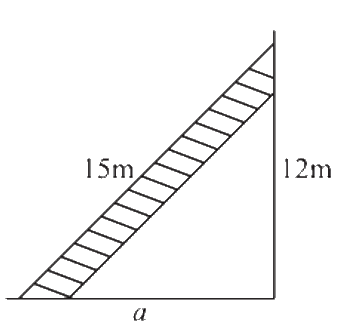### Solution

What is  known ?

Length of ladder is $$\rm{}15\, m$$ and the height of the window from the ground is $$\rm{}12\,m.$$

What is unknown?

The distance of the foot of the ladder from the wall.

Reasoning:

This question is based on the concept of right – angled triangle. As it is clear from the figure
that the ladder is kept slanted on the wall so, consider length of the ladder as hypotenuse
i.e.: $$AB =\rm{}15\,m$$ and as it is kept slanted on the wall so, we consider wall as perpendicular
i.e.: $$AC = \rm{}12\, m.$$ Now, you must find out the distance of the foot of the ladder from the wall
i.e.: $$BC = a.$$ Now by applying Pythagoras theorem in triangle $$ABC,$$ we can find out $$BC.$$

For better visual understanding draw a right-angled triangle consider ladder as hypotenuse, wall as perpendicular and distance between the foot of the ladder and wall as base.

Steps:

Given, Length of ladder $$AB = \rm{}15\, m$$

Length of wall $$AC = \rm{}12\, m$$

To find $$(BC) =$$ distance of the foot of the ladder from the wall.

According to Pythagoras theorem,

\begin{align}&{{\left(\text{Hypotenuse} \right)}^2} \\ &= {\rm{ }}{{\left(\text {Perpendicular} \right)}^2} + {\rm{ }}{{\left( \text{Base} \right)}^2} \end{align}

\begin{align} {{\left( {AB} \right)}^2} &= {{\left( {AC} \right)}^2} + {{\left( {BC} \right)}^2}\\{{\left( {15} \right)}^2} &={{\left( {12} \right)}^2} + {{\left( a \right)}^2}\\225 &= 144 + {{\left( a \right)}^2}\\225-144 &= {{\left( a \right)}^2}\\81 &= {a^2}\\a &= 9 m\end{align}

Therefore, the distance of the foot of the ladder from the wall is $$\rm{}9 \,m.$$

Useful Tip:

Whenever you encounter problem of this kind, it is better to understand its visually.

## Chapter 6 Ex.6.5 Question 4

Which of the following can be the sides of a right triangle?

(i) $$\rm{}2.5\, cm, \rm{}6.5\,cm, \rm{}6\,cm$$

(ii) $$\rm{}2\,cm, \rm{}2\, cm, \rm{}5\, cm.$$

(iii) $$\rm{}1.5\,cm, \rm{}2\,cm, \rm{}2.5\, cm.$$

In the case of right-angled triangles, identify the right angles.

### Solution

What is  known?

Sides of the triangles.

What is unknown?

Given sides are the sides of right-angled triangle or not.

Reasoning:

This question is very simple. Consider the greater side as the hypotenuse and if the square of hypotenuse is equal to the sum of square of other two sides then these are the sides of a right - angled triangle.

Steps

(i)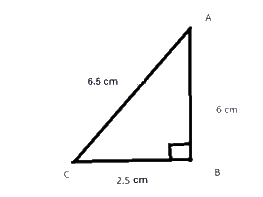\begin{align}&{{\left( \text{Hypotenuse} \right)}^2} \\&= \rm{{ }}{{\left(\text {Perpendicular} \right)}^2} + {\rm{ }}{{\left( \text{Base} \right)}^2} \end{align}

\begin{align}{{\left( {6.5} \right)}^2} &= \rm{{ }}{{\left( 6 \right)}^2} + {{\left( {2.5} \right)}^2}\\42.25{\rm{ }} &= \rm{{ }}36 + {\rm{ }}6.25\\42.25{\rm{ }} &= \rm{{ }}42.25\\ L.H.S &= R.H.S\end{align}

Therefore, given sides are of the right-angled triangle.

Right angle lies opposite to the greater side.

(ii)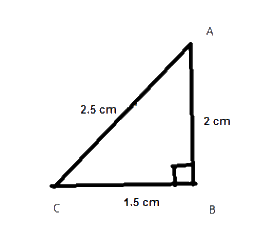\begin{align}&{{\left( \text{Hypotenuse} \right)}^2} \\&= \rm{{ }}{{\left(\text {Perpendicular} \right)}^2} + {\rm{ }}{{\left( \text{Base} \right)}^2} \end{align}

\begin{align} {{\left( 5 \right)}^2} &= \rm{{ }}{{\left( 2 \right)}^2} + {\rm{ }}{{\left( 2 \right)}^2}\\25{\rm{ }} &= \rm{{ }}4{\rm{ }} + {\rm{ }}4\\25{\rm{ }}& \ne {\rm{ }}8\end{align}

Therefore, the given sides are not of a right- angled triangle.

(iii)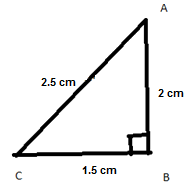\begin{align}&{{\left( \text{Hypotenuse} \right)}^2} \\&= \rm{{ }}{{\left(\text {Perpendicular} \right)}^2} + {\rm{ }}{{\left( \text{Base} \right)}^2} \end{align}

\begin{align} {{\left( {2.5} \right)}^2} &= \rm{{ }}{{\left( 2 \right)}^2} + {\rm{ }}{{\left( {1.5} \right)}^2}\\6.25{\rm{ }} &= \rm{{ }}4{\rm{ }} + {\rm{ }}2.25\\6.25{\rm{ }} &= \rm{{ }}6.25\\L.H.S&= R.H.S\end{align}

Therefore, given sides are of a right-angled triangle. Right angle lies on the opposite to the
greater side.

Useful Tip:

Whenever you encounter problem of this kind, it is best to think of the Pythagoras property
that if the square of hypotenuse or greater side is equal to the sum of square of other two sides then it is a right- angled triangle.

## Chapter 6 Ex.6.5 Question 5

A tree is broken at a height of $$\rm{}5\, m$$ from the ground and its top touches the ground at $$\rm{}12\, m$$ from the base of the tree. Find the original height of the tree.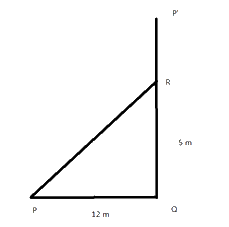### Solution

What is known?

Height of the point where the tree broke from the ground is $$5 \rm \,m$$ and distance between the base of the tree and the top of the tree when it touches the ground is $$\rm{}12\, m$$

What is unknown?

Original height of the tree..

Reasoning:

This question is also based on the concept of right-angled triangle and Pythagoras theorem. Suppose $$\rm{}P’Q$$is the height of the tree, as it is mentioned in the question that the tree is broken at a height of $$\rm{}5\,m$$ from the ground. suppose tree is broken from $$R$$ So, consider $$RQ$$ as perpendicular and $$PR$$ as broken part of the treeand, as hypotenuse. Remember, length of $$PR$$ i.e. broken part of the tree will remain same as $$P’R.$$ Now, a right – angled triangle $$PQR$$ is formed, apply Pythagoras theorem and find the length of broken part i.e. $$PR$$. As we must find out the original height of the tree for this, add the length of the broken part and the length where it broke i.e. $$QR.$$

For better visual understanding draw a right-angled triangle and visualise all the parts.

Steps

Let $$P’Q$$ represents the original height of the tree before it broken at $$R$$ and $$RP$$ represents the broken part of the tree.

Triangle $$PQR$$ is right angled at $$Q$$. So, in this triangle, according to Pythagoras theorem,

\begin{align}&{{\left( \text{Hypotenuse} \right)}^2} \\&= \rm{{ }}{{\left(\text {Perpendicular} \right)}^2} + {\rm{ }}{{\left( \text{Base} \right)}^2} \end{align}

\begin{align} {{\left( {PR} \right)}^2} &={{\left( {RQ} \right)}^2} +{{\left( {PQ} \right)}^2}\\{{\left( {PR} \right)}^2} &= \rm{{ }}{{\left( 5 \right)}^2} + {\rm{ }}{{\left( {12} \right)}^2}\\{{\left( {PR} \right)}^2} &= \rm{{ }}25 + 144\\{{\left( {PR} \right)}^2} &= \rm{{ }}169\\PR{\rm{ }} &= \rm{{ }}13{\rm{ }}m\end{align}

Thus, the original height of the tree

\begin{align}&= PR + RQ \\&= \rm{}13\, m + 5\, m\\&= \rm{}18\,\rm{m} \end{align}
Thus, the original height of the tree is $$\rm{}18\, m.$$

Useful Tip:

Whenever you encounter problem of this kind, it is best to think of the concept of a right-angled triangle.

## Chapter 6 Ex.6.5 Question 6

Angles $$Q$$ and $$R$$ of a $$\Delta\,PQR$$ are $$25^\circ$$ and $$65^\circ$$Write which of the following is true:

(i) $$PQ^2 + QR^2 = RP^2$$

(ii) $$PQ^2 + RP^2 = QR^2$$

(iii) $$RP^2 + QR^2 = PQ^2$$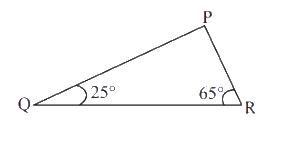### Solution

What is known?

Measure of two angles of a triangle i.e. $$25°$$and $$65°.$$

What is the unknown?

Which option is correct.

Reasoning:

This question is straight forward, in this question it is clear from the figure two angles of a triangle are given and we must find out the third angle by using angle sum property i.e. the sum of three interior angles of a triangle is $$180^\circ$$ By using this property, we got the value of third angle i.e. $$P = 90^\circ$$ that means side opposite to $$P$$ is hypotenuse i.e. $$QR$$. As one of the angles is $$90^\circ$$ that means it is a right-angled triangle and the square of hypotenuse is equal to the sum of square of other two sides.

Steps:

We know that, sum of interior angles of a triangle is $$180^\circ$$.

\begin{align}\angle P + \angle Q + \angle R &= 180^\circ \\\angle P + 25^\circ  + 65^\circ  &= 180^\circ \\\angle P + 90^\circ  &= 180^\circ \\\angle P &= 180^\circ  - 90^\circ \\\angle P &= 90^\circ \end{align}

Thus, triangle $$PQR$$ is a right angled at $$P$$

Therefore, by Pythagoras theorem

\begin{align}{(P)^2} + {(B)^2} &= {(H)^2}\\{(QP)^2} + {(PR)^2} &= {(QR)^2}\end{align}

Hence, option (ii) is correct.

Useful Tip:

Whenever you encounter problem of this kind, it is best to think of the Pythagoras property if one of the three angles is $$90^\circ$$ then the square of hypotenuse or greater side is equal to the sum of square of other two sides.

## Chapter 6 Ex.6.5 Question 7

Find the perimeter of the rectangle whose length is $$\rm{}40\, cm$$ and diagonal is $$\rm{}41\,cm$$.

### Solution

What is known?

Length of rectangle is given $$\rm{}40\, cm$$ and diagonal is $$\rm{}41\,cm.$$

What is unknown?

Reasoning:

This question is based on the two concepts i.e. the concept of rectangle and Pythagoras theorem. For better understanding of this question understand it with the help of a figure.

As it is mentioned in the question suppose there is a rectangle $$ABCD$$ and whose length is given $$\rm{}40\,cm.$$ As, we know the opposite sides of a rectangle are equal if $$AB = \rm{}40$$$$\rm\,cm$$ that means side opposite to $$AB$$ i.e. $$CD$$ will also be $$\rm{}40\, cm.$$ Now, one of the diagonals of a rectangle is given $$AC = 41\,\rm\,cm$$ it divides the rectangle into two right-angled triangles. Now, you can apply Pythagoras theorem and find the third side i.e. breadth of the rectangle. Now, you have the measure of both the sides of a rectangle i.e. length and breadth, you can easily find out the perimeter of the rectangle.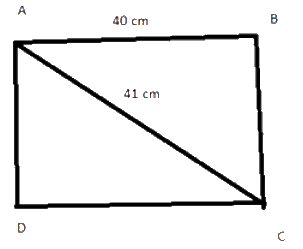Steps:

Given,in rectangle $$ABCD,AB$$ and $$CD$$ are the lengths of the rectangle.

$$AB =\rm{}40\,cm,$$ $$CD = \rm{}40\,cm$$ and $$AC$$ is the diagonal

Therefore, $$AC = \rm{}41\,cm$$

Let breadth of rectangle be $$x$$.

Now, in triangle $$ADC,$$ by Pythagoras theorem

\begin{align}&\left( \text{Hypotenuse} \right)^2 \\&= \rm{{ }}{{\left(\text {Perpendicular} \right)}^2} + {\rm{ }}{{\left( \text{Base} \right)}^2} \end{align}

\begin{align} {{\left( {AC} \right)}^2} &= {{\left( {DC} \right)}^2} + {{\left( {AD} \right)}^2}\\{{\left( {41} \right)}^2} &= {{\left( {40} \right)}^2} +{{\left( x \right)}^2}\\1681 &= 1600{\rm{ }} + {{\left( x \right)}^2}\\1681-1600 &= {{\left( x \right)}^2}\\{x^2} &= 81\\{\rm{or}} \,x &= \rm{{ }}9{\rm{ }}cm\end{align}

Therefore, breadth of rectangle $$= \rm{}9\,cm$$

Now, we know that

Perimeter of rectangle

\begin{align} &= \rm{{ }}2\left( {l{\rm{ }} + {\rm{ }}b} \right)\\&= {\rm{ }}2{\rm{ }}\left( {40{\rm{ }} + {\rm{ }}9} \right)\\& = {\rm{ }}2\left( {49} \right)\\& = {\rm{ }}98{\rm{ }}\rm\,cm\end{align}

Hence, the perimeter of rectangle is $$98\rm\,cm$$

Useful Tip:

Whenever you encounter problems of this kind, remember the Pythagoras property of right -angled triangle and the formulas related to rectangle.

## Chapter 6 Ex.6.5 Question 8

The diagonals of a rhombus measure $$\rm{}16\,cm$$ and $$\rm{}30\, cm.$$ Find its perimeter.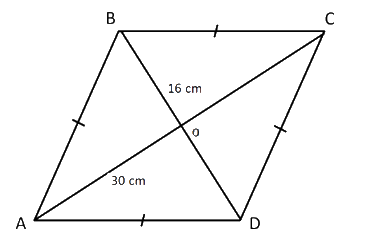### Solution

What is  known?

Diagonals of rhombus are given as $$\rm{}16\,cm$$ and $$\rm{}30\,cm.$$

What is unknown?

Perimeter of rhombus.

Reasoning:

This question is also based on the two concepts i.e. the concept of rhombus  and Pythagoras theorem. For better understanding of this question understand it with the help of a figure.

As it is mentioned in the question suppose there is a rhombus $$ABCD$$ and length of whose diagonals are given as $$16 \rm \,cm$$ and $$\rm{}30\,cm.$$. As, we know the diagonals of a rhombus bisect each other at $$90^\circ$$ i.e. at $$O$$. Now, $$O$$ divides $$DB$$ and $$AC$$ into two equal parts i.e. $$OD$$ is half of $$DB$$ and $$OC$$ is half $$AC.$$ Now, you can apply Pythagoras theorem in triangle $$DOC$$ and get the measure of side $$DC.$$ Now, the side of rhombus is known, you can easily find the perimeter of rhombus.

Steps

Given, $$AC$$ and $$BD$$ are the two diagonals of the rhombus.

$$AC = \rm{}30\,cm,$$ $$BD =\rm{}16\,cm$$

Since, the diagonal of a rhombus bisect each other at $$90^\circ.$$

Therefore,

\begin{align}OD &= \frac{{DB}}{2} = \frac{{16}}{2} = 8\;\rm{cm}\\OC &= \frac{{AC}}{2} = \frac{{30}}{2} = 15\;\rm{cm} \end{align}

Now, in right angled triangle$$\Delta \,DOC,$$

\begin{align}&\left( \text{Hypotenuse} \right)^2 \\&= \rm{{ }}{{\left(\text {Perpendicular} \right)}^2} + {\rm{ }}{{\left( \text{Base} \right)}^2} \end{align}

\begin{align} {{\left( {DC} \right)}^2} &= {{\left( {OD} \right)}^2} + {{\left( {OC} \right)}^2}\\{{\left( {DC} \right)}^2} &= \rm{{ }}{{\left( 8 \right)}^2} + {\rm{ }}{{\left( {15} \right)}^2}\\{{\left( {DC} \right)}^2} &= \rm{{ }}64{\rm{ }} + {\rm{ }}225\\{{\left( {DC} \right)}^2} &= {\rm{ }}289\\DC{\rm{ }} &= \rm{{ }}17{\rm{ }}\,cm\end{align}

\begin{align}\text{Perimeter of rhombus}&=\rm{}4\,\times \,{side}\\&=\rm{} 4 \,\times \,17 \\&= \rm{}68\, \rm{cm}\end{align}

Thus, the perimeter of rhombus is $$\rm{}68\,cm.$$

Useful Tip:

Whenever you encounter problem of this kind, it is best to think of the properties related to rhombus.

Related Sections
Related Sections
Instant doubt clearing with Cuemath Advanced Math Program